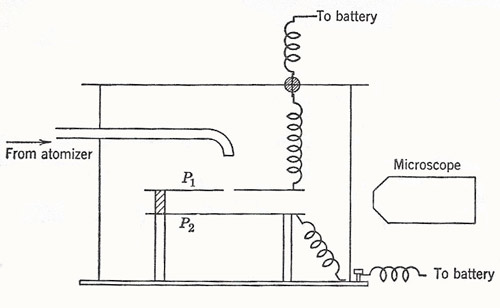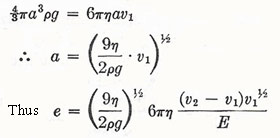A# charge on the electronFigure 1. A schematic diagram of Millikan's oil-drop apparatus. Motion of the drops is observed between the plates P1 and P2 under the combined electric and gravitational fields.

In 1897 Townsend studied the gases and vapors liberated at the electrodes in electrolysis. Not all the charge is given up at the electrodes; some is carried out on small droplets. Townsend assumed that each ion resided on a droplet. He measured (1) the total charge Q in a sample of the vapor; (2) the total mass M of the vapor, (3) the radius a and hence the mass m of a droplet by observing the fall of a cloud of the vapor under gravity, and making use of Stokes's law:and he found a value of about 3 × 10-10 electrostatic unit (esu) (≈ 10-19 coulomb (C).

In 1898 J. J. Thomson used a method similar to Townsend's. The ions were produced this time by X rays, and an ionization current density I was measured: I = ne(u + v)E, where u and v are the mobilities of positive and negative ions (i.e. the velocities in unit electric field) and n is the number of ion pairs per unit volume. Some early experiments by Rutherford had already measured the velocities of ions, and so this gave ne (= Q). Thomson obtained various values for e in the range (5.5 to 8.4) × 10-10 esu (= 1.8 to 2.8 × 10-19 C). In 1903 H. A. Wilson performed the prototype of Millikan's method. Wilson formed a cloud of electrically charged drops by an expansion method. He then measured the rate of fall of the upper edge of the cloud under gravity, and under gravity plus an electric field E. We have, for a given drop carrying one electronic charge,Also, from the first equationBy observing the top of the cloud only, the multiply charged drops are not included. Wilson found e = 3.1 × 10-10 esu (= 1.0 × 10-19 C). In 1910 Robert Millikan, by observation of individual drops, found e = 4.65 × 10-10 esu (= 1.55 × 10-19 C), with good consistency (± 0.1 × 10-1 esu). He also observed sudden changes in the speed of drops under an electric field and could verify that the change of charge corresponding to the capture of an individual ion was equal to e or some small multiple of it. Millikan later used oil drops to overcome the difficulty of evaporation and hence change of size of a drop while under observation (Figure 1).

The apparent value of e began to change as very small drops were used. The led to the discovery that η was not strictly a constant. The final result of the experiments was

e = (4.807 ± 0.005) × 10-10 esu = 1.60 × 10-19 C.